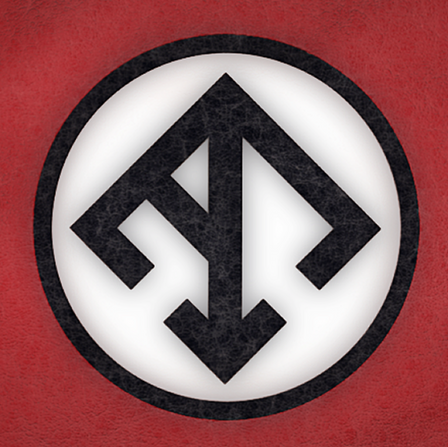### How can I create PNG image files from STL files?

•Mark Harrison

4 years ago

I need to add some simple image renders of STL files to a document. I currently open the STL files in Preview or one of the slicers and grab a screen shot.

Is there an easier or automatic way to generate PNG images from STL files on a Mac?The answer below sounds pretty good, so I'll just comment that Google turns up a number of options for generating *postscript* files from STL, and you should be able to convert those to other image formats. I'm not sure how lossy that transition would be (and it'll vary by tool), but figured I'd mention it in case someone comes here looking for e.g. a non-Mac solution...

•4 years ago

If you have OpenSCAD installed, this shell script will generate 100x100 pixel PNG images for each STL file in your current directory.

``for i in *.stl; do  T=__tmp__$$i b=`basename$$i`  echo import\(\"$$i\"\)\; >$$T  /Applications/OpenSCAD.app/Contents/MacOS/OpenSCAD -o $$b.png --imgsize=100,100$$T  rm \$Tdone``

Credit to 0scar for pointing out STL files can be imported into OpenSCAD.

Update: This code does the same, and generates an html file with annotated images of the files rendered. When I printed a batch of spare parts for my 3D printer I made a hardcopy and stuck it in the box so I could identify the parts later.

``n=-1H=00-catalog.htmlecho >$$H ''echo >>$$H '  <tr>'for i in $$*; do n=`expr$$n + 1`  if test \$n = 4; then      n=0      echo >>\$H '  </tr>'      echo >>\$H '  <tr>'  fi  echo \$i  T=__tmp__\$i  B=`basename $$i .stl` echo import\(\"$$i\"\)\; >$$T /Applications/OpenSCAD.app//Contents/MacOS/OpenSCAD -o$$B.png --imgsize=200,200 $$T echo >>$$H  echo >>$$H ' '$$i'<br><img src="'\$B'.png"></td>'  rm $$Tdoneecho >>$$H '  </tr>'echo >>\$H '</table>'``